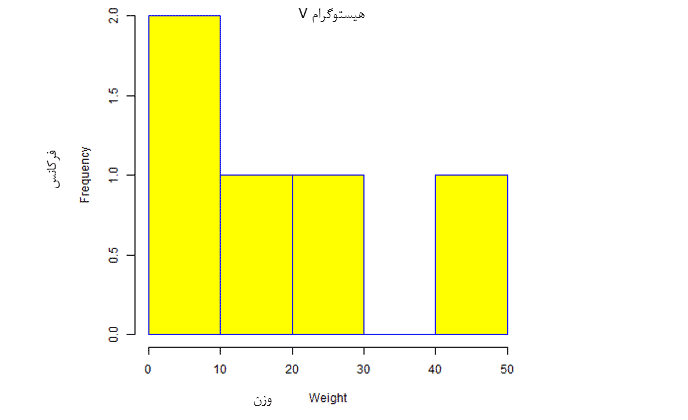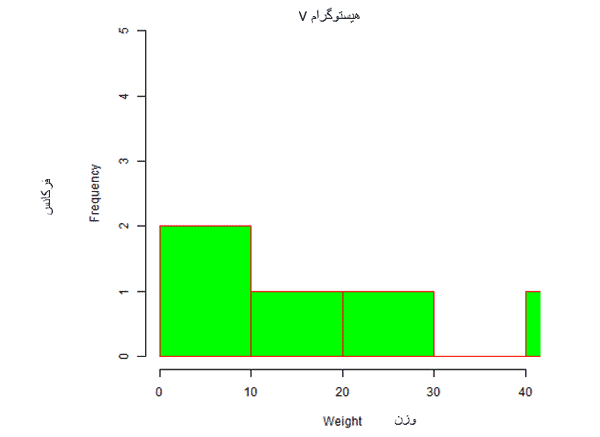# blog posts# A histogram represents the frequencies of the values ​​of a variable that are placed in a range. The histogram is similar to a bar chart, but the difference is that groups of values ​​are displayed in continuous ranges.

Each column in the histogram represents the height of the number of values ​​in the range.

In the R programming language, the histogram uses the hist () function to create histograms. This function takes a vector as an input and uses some parameters to draw a histogram.

## Syntax

The basic syntax for creating a histogram using R is as follows:

hist (v, main, xlab, xlim, ylim, breaks, col, border)

Parameters used in the above coding; as follows:

• v is a vector that contains the numerical values ​​used in the histogram.
• main represents the title of the chart.
• col is used to adjust the color of columns.
• xlab is used to provide an x-axis explanation.
• ylab is used to explain the y-axis.
• xlim is used to specify the range of values ​​on the x-axis.
• ylim is used to specify the range of values ​​on the y-axis.
• breaks are used to express the width of each column.

## Example

A simple histogram is created using the input vector, col and border parameters.

The following script; The histogram generates and stores the current R in the currently working directory.

# Create data for the graph.

v <- c (9,13,21,8,36,22,12,41,31,33,19)

# Give the chart file a name.

png (file = “histogram.png”)

# Create the histogram.

hist (v, xlab = “Weight”, col = “yellow”, border = “blue”)

# Save the file.

dev.off ()

When we run the above code; The following result is obtained:## Range of X and Y values

To specify the range of permissible values ​​on the X axis and the Y axis; We can use the xlim and ylim parameters.

Using distances, the width of each column can be determined.

# Create data for the graph.

v <- c (9,13,21,8,36,22,12,41,31,33,19)

# Give the chart file a name.

png (file = “histogram_lim_breaks.png”)

# Create the histogram.

hist (v, xlab = “Weight”, col = “green”, border = “red”, xlim = c (0,40), ylim = c (0,5),

breaks = 5)

# Save the file.

dev.off ()

When we run the above code; Creates the following histograms result: